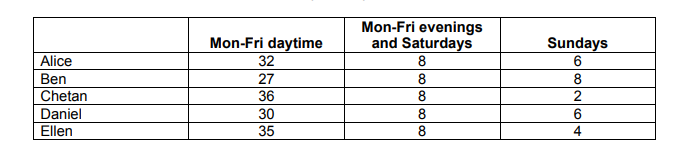# IMAT 2011 Q11 [Printing Company]

Employees at a printing company are paid a basic rate of €11 per hour during the day Monday to Friday. During weekday evenings and on Saturdays they are paid at one and a half times the basic rate and on Sundays, they are paid at double the basic rate.
The table below shows the hours worked by employees last week:Which employee earned most last week?

A. Alice

B. Ben

C. Chetan

D. Daniel

E. Ellen

1 Like

This question can be solved by using simple algebra. One thing we could take advantage of is common, repeating numbers. As it seems like we will have to add and multiply many numbers, we might want to recognize the numbers we can get rid of right from the start.

The middle column on the table states that all workers worked 8 hours during Mon-Fri evenings and Saturday. Because all workers earn the same and worked the same amount of hours (8), we can ignore the middle column completely.

Another thing to remember is that because all workers earn the same hourly rate, we will only need to multiply the third column by 2x11 (double the amount of the hourly rate) but not the first column by 11 (the hourly fee) because we compare the third column to our default - the first column.

Thus, all we need to do in this question is to add the hours from the first column to the third column, doubling the third column as we consider 1 hour of work on Sunday to 2 hours of work during the weekday.

Alice = 32 + 6x2 = 44
Ben = 27 + 8x2 = 43
Chetan = 36 + 2x2 = 40
Daniel = 30 + 6x2 = 42
Ellen = 35 + 4x2 = 43

Alice earned the most last week.

2 Likes

Thank you Ari for your constant help for students.
I wonder why we did not also multiply the first column by 11 in order to find the total amount of money earned during these hours.
For instance, (3211)+ (226)
so we can add up things with the same unit, like euros and euros, not hours and euros.